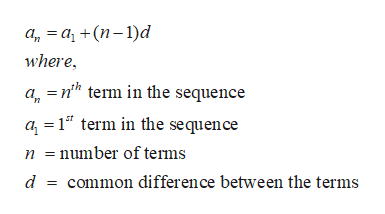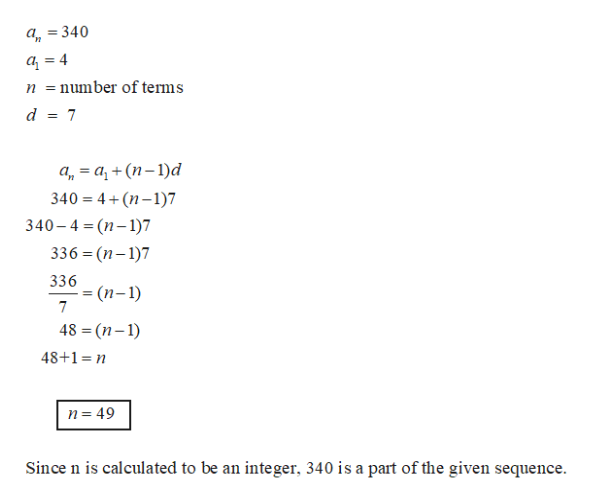# Consider the sequence 4, 11, 18, 25, 32, ....a. Is 340 a member of the sequence?b. Is 738 a member of the sequence?

Question

Consider the sequence 4, 11, 18, 25, 32, ....

a. Is 340 a member of the sequence?

b. Is 738 a member of the sequence?

check_circleExpert Solution
Step 1

It can be observed that the given sequence has a constant difference of 7 between the successive terms. Hence it is an arithmetic progression.help_outlineImage Transcriptioncloseа, 3 а, +(п-1)d where, =nh term in the sequence а, a 1 term in the sequence number of tems d common difference between the terms fullscreen
Step 2

Substitute the term to be tested in the above formula and calculate n.

If n turns out to be an intege...help_outlineImage Transcriptionclosea, =340 4 = number of terms d 7 а, — а, + (п-1)d 340=4+(n-1)7 340-4 %3D (п-1)7 336 (n-1)7 336 (п-1) 48 (n-) 48+1 n n 49 Since n is calculated to be an integer, 340 is a part of the given sequence fullscreen

### Want to see the full answer?

See Solution

#### Want to see this answer and more?

Solutions are written by subject experts who are available 24/7. Questions are typically answered within 1 hour*

See Solution
*Response times may vary by subject and question
Tagged in

### Other